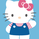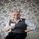# ------ Chop + Vdub FX SniperVX3-- for day trading -- use 1-5 min

4106 views
4106
Hello everyone,

I've adapted two scripts chop + vdub for day trading use. Use 1-2-3-4-5 minutes for test.
Back test for this Week only!!! Change the timestamps for using in the next week etc!!
```//@version=2
//╭╮╱╱╭╮╭╮╱╱╭╮
//┃╰╮╭╯┃┃┃╱╱┃┃
//╰╮┃┃╭┻╯┣╮╭┫╰━┳╮╭┳━━╮
//╱┃╰╯┃╭╮┃┃┃┃╭╮┃┃┃┃━━┫
//╱╰╮╭┫╰╯┃╰╯┃╰╯┃╰╯┣━━┃  ///extended vDr
//╱╱╰╯╰━━┻━━┻━━┻━━┻━━╯
//╭━━━┳╮╱╱╱╱╱╱╱╭╮
//┃╭━╮┃┃╱╱╱╱╱╱╱┃┃
//┃┃╱╰┫╰━┳━━┳━╮╭━╮╭━━┫┃
//┃┃╱╭┫╭╮┃╭╮┃╭╮┫╭╮┫┃━┫┃
//┃╰━╯┃┃┃┃╭╮┃┃┃┃┃┃┃┃━┫╰╮
//╰━━━┻╯╰┻╯╰┻╯╰┻╯╰┻━━┻━╯
//━╯
// http://www.vdubus.co.uk/
strategy(title='------ Chop + Vdub FX SniperVX3', shorttitle='----Chop+Vdub_FX_SniperVX3', overlay=true, pyramiding=1, initial_capital=1000, currency=currency.USD)

//Candle body resistance Channel-----------------------------//
len = 34
src = input(close, title="Candle body resistance Channel")
out = sma(src, len)
last8h = highest(close, 13)
lastl8 = lowest(close, 13)
bearish = cross(close,out) == 1 and falling(close, 1)
bullish = cross(close,out) == 1 and rising(close, 1)
channel2=input(false, title="Bar Channel On/Off")
ul2=plot(channel2?last8h:last8h==nz(last8h)?last8h:na, color=black, linewidth=1, style=linebr, title="Candle body resistance level top", offset=0)
ll2=plot(channel2?lastl8:lastl8==nz(lastl8)?lastl8:na, color=black, linewidth=1, style=linebr, title="Candle body resistance level bottom", offset=0)
//fill(ul2, ll2, color=black, transp=95, title="Candle body resistance Channel")

//-----------------Support and Resistance
RST = input(title='Support / Resistance length:', type=integer, defval=10)
RSTT = valuewhen(high >= highest(high, RST), high, 0)
RSTB = valuewhen(low <= lowest(low, RST), low, 0)
RT2 = plot(RSTT, color=RSTT != RSTT ? na : red, linewidth=1, offset=+0)
RB2 = plot(RSTB, color=RSTB != RSTB ? na : green, linewidth=1, offset=0)

//--------------------Trend colour ema------------------------------------------------//
src0 = close, len0 = input(13, minval=1, title="EMA 1")
ema0 = ema(src0, len0)
direction = rising(ema0, 2) ? +1 : falling(ema0, 2) ? -1 : 0
plot_color = direction > 0  ? lime: direction < 0 ? red : na
plot(ema0, title="EMA", style=line, linewidth=1, color = plot_color)

//-------------------- ema 2------------------------------------------------//
src02 = close, len02 = input(21, minval=1, title="EMA 2")
ema02 = ema(src02, len02)
direction2 = rising(ema02, 2) ? +1 : falling(ema02, 2) ? -1 : 0
plot_color2 = direction2 > 0  ? lime: direction2 < 0 ? red : na
plot(ema02, title="EMA Signal 2", style=line, linewidth=1, color = plot_color2)

//=============Hull MA//
show_hma = input(false, title="Display Hull MA Set:")
hma_src = input(close, title="Hull MA's Source:")
hma_base_length = input(8, minval=1, title="Hull MA's Base Length:")
hma_length_scalar = input(5, minval=0, title="Hull MA's Length Scalar:")
hullma(src, length)=>wma(2*wma(src, length/2)-wma(src, length), round(sqrt(length)))
plot(not show_hma ? na : hullma(hma_src, hma_base_length+hma_length_scalar*6), color=black, linewidth=2, title="Hull MA")

rangeup = close+tr
rangedown = close-tr
over() => high > rangeup and close > open
under() => low < rangedown and close < open

//============ signal Generator ==================================//
Piriod=input('720')
ch1 = security(tickerid, Piriod, open)
ch2 = security(tickerid, Piriod, close)

longCondition = crossover(security(tickerid, Piriod, close),security(tickerid, Piriod, open))
//if (longCondition )
//    strategy.entry("Vdub Long", strategy.long, when = over())

shortCondition = crossunder(security(tickerid, Piriod, close),security(tickerid, Piriod, open))
//if (shortCondition)
//    strategy.entry("Vdub Short", strategy.short, when = under())
//if(over())
//    strategy.exit("Vdub Short")
//if(under())
//    strategy.exit("Vdub Long")
//strategy.exit("Vdub Short", loss = 10)
//strategy.exit("Vdub Long", loss = 10)

strategy.entry("CBL", strategy.long, when = over() and time >= (timestamp(2016, 03, 07, 09, 30)) and time < (timestamp(2016, 03, 12, 00, 00)))
strategy.entry("CBS", strategy.short, when = under() and time >= (timestamp(2016, 03, 07, 09, 30)) and time < (timestamp(2016, 03, 12, 00, 00)))

strategy.exit(id="XVdubL",from_entry="CBL", when = under() or shortCondition, loss=20)
strategy.exit(id="XVdubS",from_entry="CBS", when = over() or longCondition, loss=20)

///////////////////////////////////////////////////////////////////////////////////////////```I don't understand why so many people on this site are creating strategies based on future data. Everyday I contemplate on leaving this site. I've wasted countless days on backtests that rely on future data. This is the only website I know that allows this to happen. Unreal